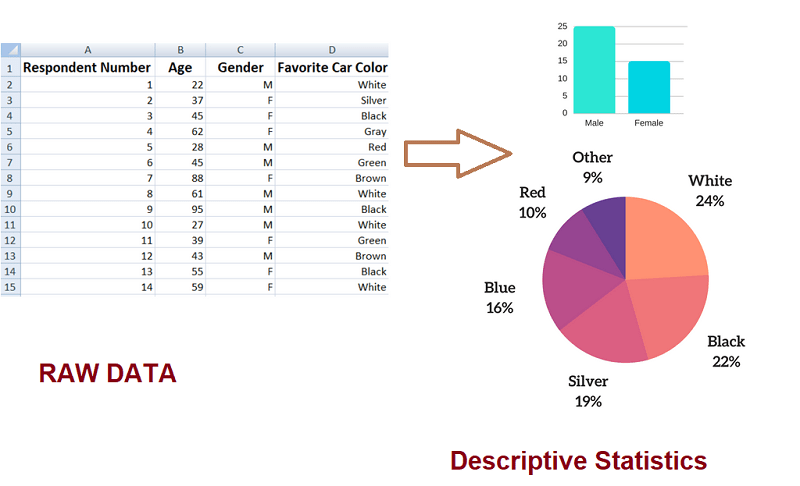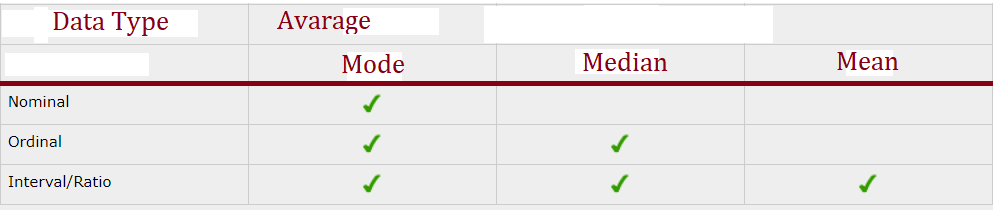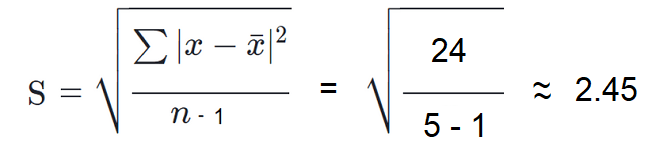# Descriptive Statistics Examples, Types and Definition

When it comes to descriptive statistics examples, problems and solutions, we can give numerous of them to explain and support the general definition and types.

Let’s first clarify the main purpose of descriptive data analysis. It’s to help you get a feel for the data, to tell us what happened in the past and to highlight potential relationships between variables.

• What is descriptive data analysis?
• The different types of descriptive statistics: explained.
• 8 examples of descriptive statistics

In the world of statistical data, there are two classifications: descriptive and inferential statistics.

In a nutshell, descriptive statistics just describes and summarizes data but do not allow us to draw conclusions about the whole population from which we took the sample.

You are simply summarizing the data with charts, tables, and graphs.

Conversely, with inferential statistics, you are using statistics to test a hypothesis, draw conclusions and make predictions about a whole population, based on your sample.

Let’s see the first of our descriptive statistics examples.

Example 1:

Descriptive statistics about a college involve the average math test score for incoming students. It says nothing about why the data is so or what trends we can see and follow.

Descriptive statistics help you to simplify large amounts of data in a meaningful way. It reduces lots of data into a summary.

Example 2:

You’ve performed a survey to 40 respondents about their favorite car color. And now you have a spreadsheet with the results.

However, this spreadsheet is not very informative and you want to summarize the data with some graphs and charts that can allow you to come up with some simple conclusions (e.g. 24% of people said that white is their favorite color).

For sure, this would be much more representative and clear than an ugly spreadsheet. And you have a plenty of options to visualize data such as pie charts, line charts, etc.

That’s the core of descriptive statistics. Note that you are not drawing any conclusions about the full population.## The 2 Main Types of Descriptive Statistics (with Examples)

Descriptive statistics has 2 main types:

• Measures of Central Tendency (Mean, Median, and Mode).
• Measures of Dispersion or Variation (Variance, Standard Deviation, Range).

1. Central Tendency

Central tendency (also called measures of location or central location) is a method to describe what’s typical for a group (set) of data.

It means central tendency doesn’t show us what is typical about each one piece of data, but it gives us an overview of the whole picture of the entire data set.

It tells us what is normal or average for a given set of data. There are three key methods to show central tendency: mean, mode, and median.

• Mean

As the name suggests, mean is the average of a given set of numbers. The mean is calculated in two very easy steps:

1. Find the whole sum as add the data together
2. Divide the sum by the total number of data

The below is one of the most common descriptive statistics examples.

Example 3:

Let’s say you have a sample of 5 girls and 6 boys.

The girls’ heights in inches are: 62, 70, 60, 63, 66.

To calculate the mean height for the group of girls you need to add the data together:

62 + 70 + 60 + 63 + 65 = 320.

Now, you take the sum (320) and divide it by the total number of girls (5): 320 / 5 = 64.

So, our mean is 64.

The best advantage of the mean is that it can be used to find both continuous and discrete numerical data (see our post about continuous vs discrete data).

Of course, the mean has limitations. Data must be numerical in order to calculate the mean. You cannot work with the mean when you have nominal data (see our post about nominal vs ordinal data).

• Mode

The mode of a set of data is the number in the set that occurs most often.

Let’s see the next of our descriptive statistics examples, problems and solutions.

Example 4:

Consider you have a dataset with the retirement age of 10 people, in whole years:

55, 55, 55, 56, 56, 57, 58, 58, 59, 60

To illustrate this let’s see table below that shows the frequency of the retirement age data.

 Retirement Age Frequency 55 3 56 2 57 1 58 2 59 1 60 1

As you see, the most common value is 55. That is why the mode of this data set is 55 years.

The mode has one very important advantage over the median and the mean. It can be calculated for both numerical and categorical data (see our post about categorical data examples).

Limitations of the mode: In some data sets, the mode may not reflect the centre of the set. In the above example, if we order the retirement age from lowest to the highest, would see that the centre of the data set is 57 years, but the mode is lower, at 53 years.

• Median

Simply said, the median is the middle value in a data set. As you might guess, in order to calculate the middle, you need:

–  first listing the data in a numerical order
– second, locating the value in the middle of the list.

Example 5:

The middle number in the below set is 26 as there are 4 numbers above it and 4 numbers below:

21, 22, 24, 24, 26, 27, 28, 29, 31.

But this was an odd set of data – you have 9 numbers. How to find the middle if you have an even set of data?

Easily – you just need to find the average of the two middle numbers.

For example, in the below dataset of 10 numbers, the average of the numbers is 26.5 (26 + 27) / 2.

21, 22, 24, 24, 26, 27, 28, 29, 31, 32

As an advantage of the median, we can say that it is less reflected by outliers and skewed data than the mean. We usually prefer the median when the data set is not symmetrical.

And to point the limitation, we should say that as the median cannot be ordered in a logical way, it cannot be calculated for nominal data.

Having trouble to remember the difference between the mode, mean, and median? Here are some hints:

• The word MOde is very like MOst (the most frequent number)
• “Mean” requires you do some arithmetic (adding all the numbers together and dividing).
• “Median” practically means “Middle” and has the same number of letters.

Having trouble to decide which measure to use when you have nominal, ordinal or interval data? The above table can help.2. Dispersion

Central tendency tells us important information but it doesn’t show everything we want to know about average values. Central tendency fails to reveal the extent to which the values of the individual items differ in a data set.

Measures of dispersion do a lot more – they complement the averages and allow us to interpret them much better.

Dispersion in statistics describes the spread of the data values in a given dataset. In other words, it shows how the data is “dispersed” around the mean (the central value).

Example 6:

Imagine you have to compare the performance of 2 group of students on the final math exam. You find that the average math test results are identical for both groups.

Is that mean the students in the two groups are performing equally? NO! Let’s see why.

Group of students A: 56, 58, 60, 62, 64
Group of students B: 40, 50, 60, 70, 80

Both of these groups have mean scores of 60.

However, in group A the individual scores are concentrated around the center – 60. All students in A have very similar performance. There is a consistency.

On the other hand, in group B the mean is also 60 but the individual scores are not even close to the center. One score is quite small – 40 and one score is very large – 80.

We can conclude that there is a greater dispersion in group B.

Note:

The study of dispersion has a key role in statistical data. If in a given country there are very poor people and very rich people, we say there is a serious economic disparity. Dispersion also is very useful when we want to find the relation between the set of data.

There are two popular measures of dispersion: standard deviation and range.

Let’s see some more descriptive statistics examples and definitions for dispersion measures.

• The Range

The range is simply the difference between the largest and smallest value in a data set. It shows how much variation from the average exists.

You might guess that low range tells us that the data points are very close to the mean. And a high range shows the opposite.

Here is the formula for calculating the range:

Range = max. value – min. value

Let’s see the next of our descriptive statistics examples.

Example 7:

If we use the math results from Example 6:

Group of students A: 56, 58, 60, 62, 64
Group of students B: 40, 50, 60, 70, 80

we easily can calculate the range:

Group A: 64 – 56 = 8

Group B: 80 – 40 = 40

You see that the data values in Group A are much closer to the mean than the ones in Group B.

A serious disadvantage of the Range is that it only provides information about the minimum and maximum of the data set. It tells nothing about the values in between.

• The Standard Deviation

Standard deviation also provides information on how much variation from the mean exists. However, the standard deviation goes further than Range and shows how each value in a dataset varies from the mean.

As in the Range, a low standard deviation tells us that the data points are very close to the mean. And a high standard deviation shows the opposite.

The standard deviation formula for a sample of a population is:Example 8:

If we use the math results in Example 6:

Group of students A: 56, 58, 60, 62, 64

The mean is 60.

Let’s find the standard deviation of the math exam scores by hand. We use simple values for the purposes of easy calculations.Now, let’s replace the values in the formula:The result above shows that, on average, every math exam score in The Group of students A is approximately 2.45 points away from the mean of 60.

Of course, you can calculate the above values by calculator instead by hand.

Note: The above formula is for a sample of a population. The standard deviation of an entire population is represented by the Greek lowercase letter sigma and looks like that:More examples of Standard Deviation, you can see in the Explorable site.

Conclusion:

The above 8 descriptive statistics examples, problems and solutions are simple but aim to make you understand the descriptive data better.

As you saw, descriptive statistics are used just to describe some basic features of the data in a study.

They provide simple summaries about the sample and enable us to present data in a meaningful way. It allows a simpler interpretation of the data.

Together with some plain graphics analysis, they form a solid basis for almost every quantitative analysis of data.

Descriptive statistics cannot, however, be used for making conclusions beyond the data we have analyzed or making conclusions regarding any hypotheses.